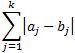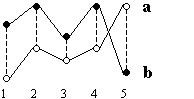﻿ City Block Distance

# City Block Distance

The City block distance between two points, a and b, with k dimensions is calculated as:The City block distance is always greater than or equal to zero. The measurement would be zero for identical points and high for points that show little similarity.

The figure below shows an example of two points called a and b. Each point is described by five values. The dotted lines in the figure are the distances (a1-b1), (a2-b2), (a3-b3), (a4-b4) and (a5-b5) which are entered in the equation above.In most cases, this distance measure yields results similar to the Euclidean distance. Note, however, that with City block distance, the effect of a large difference in a single dimension is dampened (since the distances are not squared).

The name City block distance (also referred to as Manhattan distance) is explained if you consider two points in the xy-plane. The shortest distance between the two points is along the hypotenuse, which is the Euclidean distance. The City block distance is instead calculated as the distance in x plus the distance in y, which is similar to the way you move in a city (like Manhattan) where you have to move around the buildings instead of going straight through.